Excel Len and LenN function usage(7 examples, with If,Find,Right,Subtitute in formula)

Lionsure 2019-10-25 Original by the website

Both the Len function and LenB function are used to return the length of the specified text in Excel. The Len function returns the number of characters, which counts the full-width(such as Chinese characters, Japanese characters and Korean characters) and half-width (such as numbers and letters) characters as one character; the LenB function returns the number of bytes, which counts the full-width characters as 2 bytes, half-width character as 1 byte when you have enabled the editing of a language that supports DBCS and then set it as the default language.

Len and LenB function are often combined with functions such as If, Find, Left, Mid, Right, and Substitute. For example, If + Len is used to intercept the number of characters that meet criteria, and Right + Len + find is used to intercept specified characters from the right of a string, and Len + Substitute combination counts the number of text-specified phrases in a cell.

I, Syntax of Excel Len and LenB function

1. Len function expression: LEN(Text)

2. LenB function expression: LENB(Text)

3. Description:

A. Len and LenB function return both the length of the specified text, but they differ: the Len function returns the number of characters in the specified text, it counts each half-width character(such as "number or letter") and full-width characters(such as "Chinese characters, Japanese characters and Korean characters") as one byte; the LenB function returns the number of bytes of the specified text, which counts half-width characters as one byte and full-width characters as two bytes.

B.Len function and LenB function count spaces as characters, but empty text(such as ""), they are ignored.

II, The examples of Excel Len function

(I) Return space and the length of the empty text

1. Select the cell A1, enter the formula = LEN(" "), press Enter, return 1; double-click A1, delete the space between the double quotes, press Enter, return 0; double-click A1 again, change double quotes to B1, press Enter, also return 0; the operation steps, as shown in Figure 1: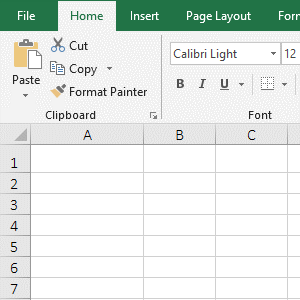Figure 1

2. Formula description:

A. The formula =LEN(" ") returns the length of the space " ", the result is 1, indicating that the Len function counts the length of the space as 1.

B. The formula =LEN("") and =LEN(B1) returns the length of the empty text "" and the empty cell, the result is 0, indicating that the Len function ignores the length of empty text and empty cells.

(II) Return the length of half-width and full-width characters

1. Select the cell B1, enter the formula =LEN(A1), press Enter, return to 28; select B2, enter the formula =LEN(A2), press Enter, return to 15; the operation steps are as shown in Figure 2: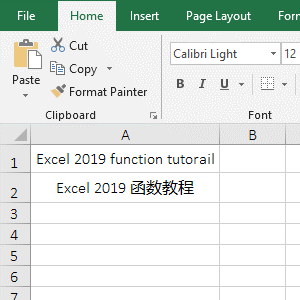Figure 2

2. Formula description:

A. Formula =LEN(A1) returns the length of text in A1. The text of A1 consists of "spaces, letters and numbers".

B. Formula =LEN(A2) returns the length of text in A2. The text of A2 consists of half-width characters("spaces, letters and numbers") and full-width characters ("Chinese characters"). These add up to 15 characters, indicating the Len function counts Both half-width and full-width characters as one character.

III, The examples of Excel LenB function

(I) Return the length of the asterisk * and question mark ?

1. Double-click the cell B1, copy the formula =LENB(A1) to B1, press Enter, return to 2; the operation steps are as shown in Figure 3: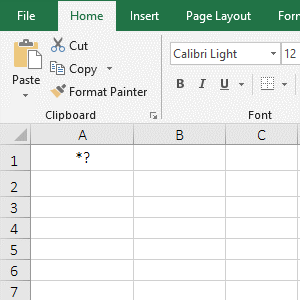Figure 3

(II) Return the length of the full-width character and half-width character

1. Double-click the cell B1, copy the formula =LENB(A1) to B1, press Enter, return to 10; select B2, enter the formula =LENB(A2), press Enter, return to 19; the operation steps are as shown in Figure 4: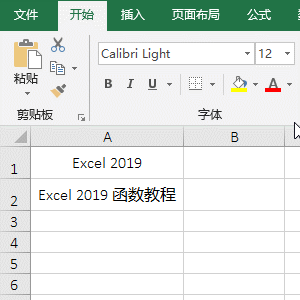Figure 4

2. "Excel 2019" in A1 has 5 letters, 4 digits and 1 space, adding up to 10; in addition to text of A1, A2 has 1 space and 4 Chinese characters, the length is 19, indicating for each Chinese character, the LenB function counts as 2 bytes, and numbers, letters, and spaces are counted as one byte, when you have enabled the editing of a language(Chinese) that supports DBCS and then set it as the default language.

IV, The application examples of Excel Len function and LenB function

(I) If Len Excel

1. If two digits are required to intercept one digit, four digits intercept two digits, and six digits intercept four digits. Double-click the cell B1, copy the formula =IF(LEN(A1)=2,LEFT(A1,1),IF(LEN(A1)=4,LEFT(A1,2),IF(LEN(A1)=6,LEFT(A1,4)))) to B1, press Enter, return 1; select B1, double-click the cell fill handle in the lower right corner of B1, then return the interception result of the remaining numbers; the operation steps are as shown in Figure 5: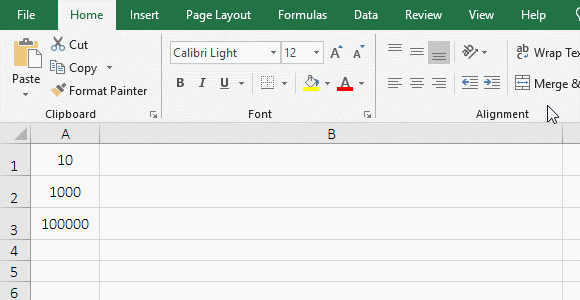Figure 5

2. Formula =IF(LEN(A1)=2,LEFT(A1,1),IF(LEN(A1)=4,LEFT(A1,2),IF(LEN(A1)=6,LEFT(A1,4)))) description:

A. LEN(A1) returns the length of the number in A1; LEN (A1)=2 is the first IF condition from the left, if LEN(A1) is equal to 2, then executes LEFT(A1,1), that is, a number is truncated from the left by using the Left function; otherwise executes IF(LEN(A1)=4,LEFT(A1,2),IF(LEN(A1)=6,LEFT(A1,4)).

B. Continue to execute, If LEN(A1)=4 is established, execute LEFT(A1,2), that is, use the Left function to intercept two digits from the left; otherwise, execute IF(LEN(A1)=6,LEFT(A1,4); the last If and so on.

(II) Right Len Find Excel

1. If you want to intercept the two words on the right. Double-click the cell B1, copy the formula =RIGHT(A1,LEN(A1)-FIND("fun",A1)+1) to B1, press Enter, return to "function tutolail"; the operation steps are as shown in Figure 6: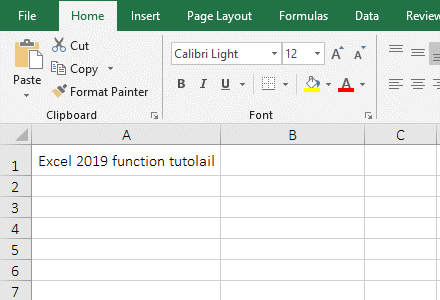Figure 6

2. Formula =RIGHT(A1,LEN(A1)-FIND("fun",A1)+1) explanation:

A. FIND("fun",A1) is used to return the starting position of the "function tutolail", the result is 12.

B. LEN(A1) is used to return the length of text in A1, the result is 28. LEN(A1)-FIND("fun",A1)+1 is used to calculate the length of the character to be intercepted, resulting in 28 - 12 + 1 = 17.

C. The formula becomes =RIGHT(A1,17), and finally the right function is used to intercept 17 characters from the right side, which is exactly the "function tutolail".

(III) Len + Subtitute function combination returns the number of specified phrases in the text.

1. If you want to count the number of a word (such as excel) in a cell. Double-click the cell A2, copy the formula =(LEN(A1)-LEN(SUBSTITUTE(A1,"excel","")))/LEN("excel") to A2, press Enter, return to 3; double-click A1, Change E to e, the value in A2 becomes 4; the operation steps are as shown in Figure 7: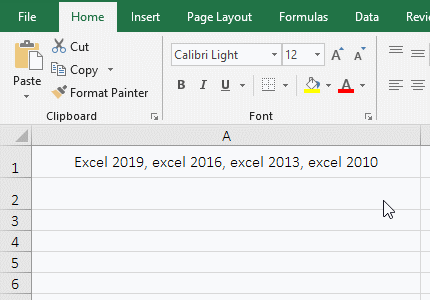Figure 7

2. Formula =(LEN(A1)-LEN(SUBSTITUTE(A1,"excel","")))/LEN("excel") explanation:

A. SUBSTITUTE (A1,"excel","") is used to replace all "excel" in A1 with a null value ""; LEN(SUBSTITUTE(A1,"excel","")) is used to return the length of all characters except "excel" in A1, the result is 28.

B. LEN(A1) is used to return the length 43 of A1, then LEN(A1)-LEN(SUBSTITUTE(A1,"excel","")) becomes 46 - 31, and the result is equal to 15, which is the number of all letters of all "excel" in A1.

C. LEN("excel") is used to return the number 5 of letters of "excel", the formula becomes 15/5, the result is equal to 3; it is worth noting that the Substitute function is case-sensitive, and "Excel" starting with an uppercase E is not Instead, the result counts 3 excels, and when E is changed to e, only 4 are counted.

(IV) The problem that the length of each Chinese(or Japanese or Korean) character is 1 by the LenB function

1. If the default language of Excel is not set to "Chinese", the LenB function counts the length of each Chinese character as 1. To solve this problem, simply set the default language of Excel to "Chinese"; the demo is shown in Figure 8: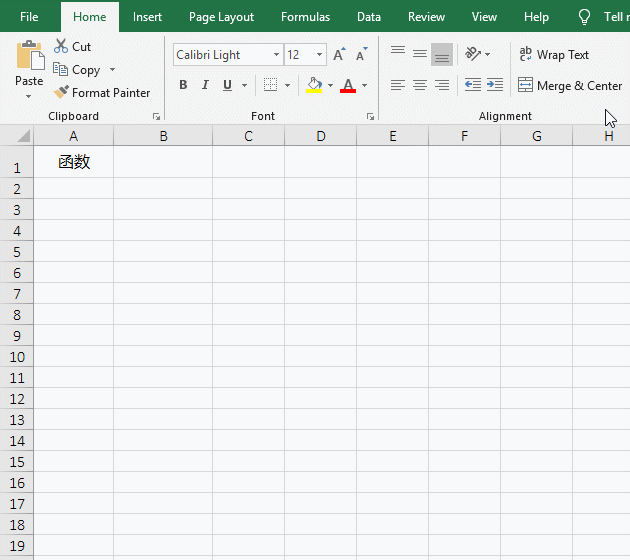Figure 8

2. After setting the default language to "Chinese", be sure to close all open Excel windows and then re-open them for the settings to take effect.